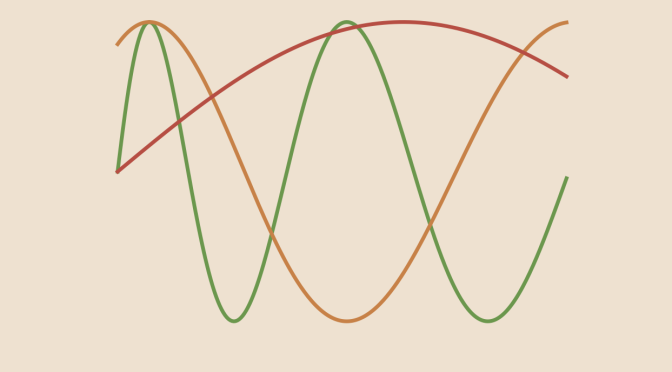# Counterexample around Arzela-Ascoli theorem

Let’s recall Arzelà–Ascoli theorem:

Suppose that $$F$$ is a Banach space and $$E$$ a compact metric space. A subset $$\mathcal{H}$$ of the Banach space $$\mathcal{C}_F(E)$$ is relatively compact in the topology induced by the uniform norm if and only if it is equicontinuous and and for all $$x \in E$$, the set $$\mathcal{H}(x)=\{f(x) \ | \ f \in \mathcal{H}\}$$ is relatively compact.

We look here at what happens if we drop the requirement on space $$E$$ to be compact and provide a counterexample where the conclusion of Arzelà–Ascoli theorem doesn’t hold anymore.

We take for $$E$$ the real interval $$[0,+\infty)$$ and for all $$n \in \mathbb{N} \setminus \{0\}$$ the real function
$f_n(t)= \sin \sqrt{t+4 n^2 \pi^2}$ We prove that $$(f_n)$$ is equicontinuous, converges pointwise to $$0$$ but is not relatively compact.

According to the mean value theorem, for all $$x,y \in \mathbb{R}$$
$\vert \sin x – \sin y \vert \le \vert x – y \vert$ Hence for $$n \ge 1$$ and $$x,y \in [0,+\infty)$$
\begin{align*}
\vert f_n(x)-f_n(y) \vert &\le \vert \sqrt{x+4 n^2 \pi^2} -\sqrt{y+4 n^2 \pi^2} \vert \\
&= \frac{\vert x – y \vert}{\sqrt{x+4 n^2 \pi^2} +\sqrt{y+4 n^2 \pi^2}} \\
&\le \frac{\vert x – y \vert}{4 \pi}
\end{align*} using multiplication by the conjugate.

Which enables to prove that $$(f_n)$$ is equicontinuous.

We also have for $$n \ge 1$$ and $$x \in [0,+\infty)$$
\begin{align*}
\vert f_n(x) \vert &= \vert f_n(x) – f_n(0) \vert \le \vert \sqrt{x+4 n^2 \pi^2} -\sqrt{4 n^2 \pi^2} \vert \\
&= \frac{\vert x \vert}{\sqrt{x+4 n^2 \pi^2} +\sqrt{4 n^2 \pi^2}} \\
&\le \frac{\vert x \vert}{4 n \pi}
\end{align*}

Hence $$(f_n)$$ converges pointwise to $$0$$ and for $$t \in [0,+\infty), \mathcal{H}(t)=\{f_n(t) \ | \ n \in \mathbb{N} \setminus \{0\}\}$$ is relatively compact

Finally we prove that $$\mathcal{H}=\{f_n \ | \ n \in \mathbb{N} \setminus \{0\}\}$$ is not relatively compact. While $$(f_n)$$ converges pointwise to $$0$$, $$(f_n)$$ does not converge uniformly to $$f=0$$. Actually for $$n \ge 1$$ and $$t_n=\frac{\pi^2}{4} + 2n \pi^2$$ we have
$f_n(t_n)= \sin \sqrt{\frac{\pi^2}{4} + 2n \pi^2 +4 n^2 \pi^2}=\sin \sqrt{\left(\frac{\pi}{2} + 2 n \pi\right)^2}=1$ Consequently for all $$n \ge 1$$ $$\Vert f_n – f \Vert_\infty \ge 1$$. If $$\mathcal{H}$$ was relatively compact, $$(f_n)$$ would have a convergent subsequence with $$f=0$$ for limit. And that cannot be as for all $$n \ge 1$$ $$\Vert f_n – f \Vert_\infty \ge 1$$.